## Wednesday, December 22, 2010

### Reflection of waves

Reflection of a wave occurs when a wave strikes an obstacle. The wave undergoes a change in direction of propagation when it is reflected.The phenomenon of reflection of waves obeys the Law of reflection where:
1. The angle of reflection is notequal to the angle of incidence.
2. The incident wave, the reflected wave and normal lie in the same plane which is perpendicular to the reflecting surface at the point of incidence.

Characteristics of reflection of waves:
1. The wavelength , speed and frequency of the reflected waves is the same as that of the incidence.
2. Angle of incidence = angle of reflection.
3. Direction of propagation of wave changes.Application of reflection of waves:

2. Makeup mirror
3. Car rear mirror and side mirror for your safety .
4. Optical fibre
5. Ultrasonic
6. Fishing with sonar.
7. Seawall to deflected the energy of the waves from the coast.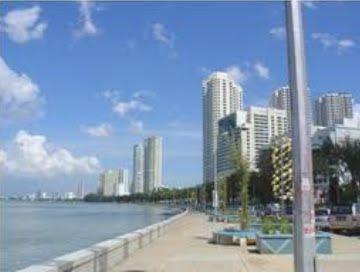Seawall

Fishing with sonar

## Monday, December 20, 2010

### Displacement- Time graph and displacement -Distance Graph for a waveThe sinusoidal graph shown is a graph of displacement, s against time , t of a load on a spring.

O is called the equilibrium position.

From a displacement-time graph for a wave, we can obtain
i) amplitude, a ,and
ii) period, T.From a displacement- distance graph for a wave, we can obtain
i) amplitude, a ,and
ii) wavelength, λ

Relationship between Speed, (v) Wavelength,(λ) and Frequency (f)

The speed of a wave is determined by its wavelength, λ, and its frequency, f , according ti the equation V= f λ

For a wave moving at constant speed, increasing the frequency will result in a decrease in the wavelength.

For a wave moving at constant speed, decreasing the frequency will result in an increase in the wavelength.

Work example:

What is the frequency of water waves with the wavelength of 3.0 cm and traveling at a speed of 1.5 cm/s ?

solution :

Step 1: write formula first
V= f λ

step 2 : Substitute all given info into formula
1.5
cm/s = f x 3.0 cm

Step 3:find unknown
f = 0.5 Hz

## Wednesday, December 8, 2010

### Wave Properties

In merupakan bab pertama dalam syllabus tingkatan 5. Murid 5 UM dan 5UKM anda diminta membuat kuiz yang disediakan. Sebarang pertanyaan boleh ditulis pada Cbox atau hantar ke Facebook saya.

1. Waves are produced by vibrating systems.Vibrating systems

2. Waves transfer energy. Waves that travel through a medium transfer energy without transferring matter.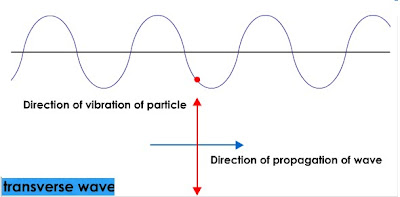3. In transverse waves, the direction of waves propagation is perpendicular to the direction of vibration of the particles.
Example: water waves, light waves and electromagnetic waves.4. In longitudinal waves, the direction of wave propagation is parallel to the direction of vibration of the particles.
Longitudinal waves need a medium to propagate. This type of waves cannot propagate through a vacuum.
Example: a sound wave.5. A wavefront is an imaginary line representing all parts of a wave in which particles are vibrating in the same phase and have the same distance from the source.
The direction of propagation of waves is always perpendicular to the wavefront.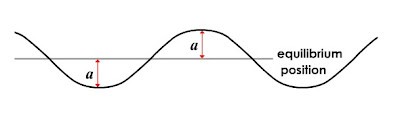6. Amplitude, a, is the maximum displacement from the equilibrium position.

7. Period, T, is the time required to make one complete oscillation.

8. Frequency, f, is the number of complete oscillations made in one second.

9. Wavelength, is defined as the distance between two consecutive points that are in phase.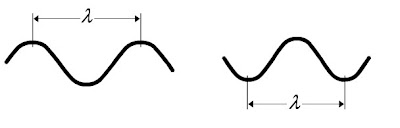( i ) For a transverse wave, wavelength can be measured as the distance from one crest and also the distance from one trough to the next trough.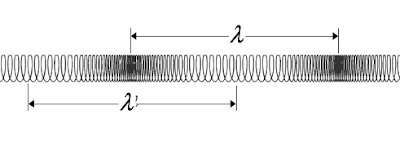(ii ) For a longitudinal wave, wavelength can be measured as the distance between
two consecutive compressions or two consecutive rarefactions.## Friday, December 3, 2010

### Gred SPM mulai 2009

Berikut merupakan gred SPM yang mula diperkenalkan mulai 2009.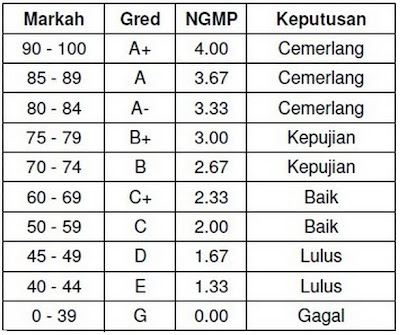SPM Candidates All the Best!